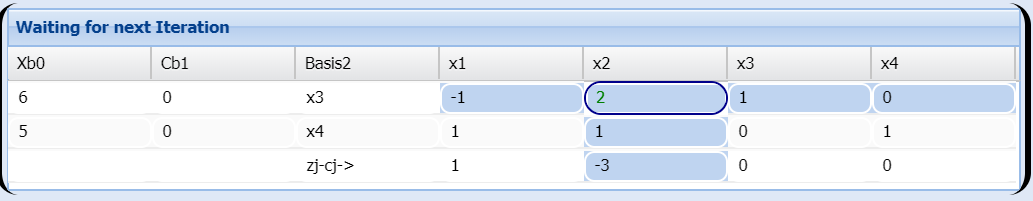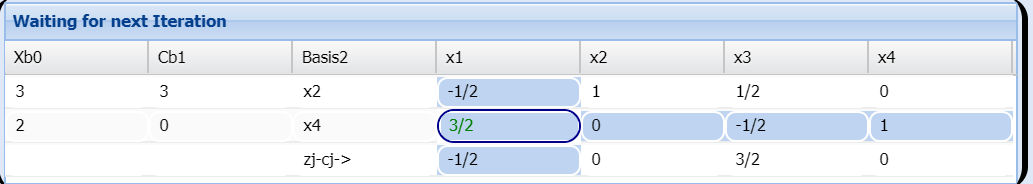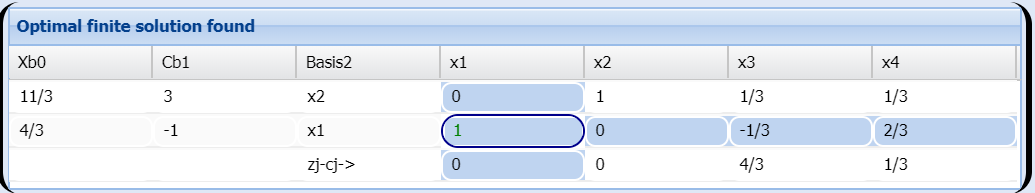# The Pivot element and the Simplex method calculations

### Basic concepts and principles

The basis of the simplex algorithm is that there is not need to calculate the inverse of matrix B to calculate the extreme points of feasible region (Remember: B is an square submatrix of A with rank m).

Instead this, a serie of calculations lead to an element of matrix A called "Pivot element" and it is used to iterate through other simple calculations, from one extreme point to the next one, testing for each one of them the optimality criterion, until one of these four cases holds.

### Simplex Algorithm and two-phase Method possible Outputs

1) The optimality criterion is fulfilled in one extreme point, in this case finite optimal solution holds. Example here

2) The optimality criterion holds, but there are infinite points also holds, this is the case with infinite solutions. Example here

3) Unbounded Solution: when the objective function can grow whatever we want within the feasible region. Example here

4) We can not continue iterating and not reached the criterion of optimality. At this case constraints are inconsistent and the problem has no solution Example here.

### Extended theory

As it was said, to perform the calculations, in practice it is not necessary to invert the B matrix (remember that B is a square submatrix A of mxm and rank m), we create a table, called Tabeau for each iteration of the algorithm in which the calculations are much easier to do than to explain

The Simplex Tableau is constructed as follows:
 Base x1 ... xi ... xm
 Xb β1 ... βi ... βm
 Cb Cb1 ... Cbi ... Cbm
 a1 ... ak ... an y11 ... y1k ... y1n ... ... ... ... ... yi1 ... yik ... yin ... ... ... ... ... ym1 ... ymk ... ymn z1-c1 ... zk-ck ... zn-cn
In red we've left the pivot element that we will explain below

In this Simplex tableau, if there is an mxm identity submatrix of A, the vector Xb would be an extreme point that allows us to begin the iterations of the algorithm, is for him to calculate the $z_{j} - c_{j}$.
Vector Xb is the extreme point that allows us to initiate the iterations of the algorithm (variables in the base), vector CB , está formado by the elements of vector C that are at that time in the base, it is for him that we calculate the $z_{j} - c_{j}$. Remember $z_{j} = X_{B}a_{j}$ or $z_{j} = C^t_{B}B^{-1}a_{j}$ and B is in this step an identity matrix as suposse at the begininig.

Once calculated, we check if optimality criterion hold, ie we test

$c_{j} - z_{j} \geq 0, \; \forall j$

In case of non-compliance, we take the less s index of j's that makes

$s = min \{ k: \,\ c_{k} - z_{k} < 0 \}$

ie, we take the minimum of negative from $c_{k} - z_{k}$.

Now, with this index s, we find the called the pivot element. To do this, divide each coordinate i of the vector Xb by its coordinate column vector yis and calculate the minimal of positive this will be our r index, ie we calculate

$r = min \{ i : \frac {x_i}{y_{is}}, y_{is} \geqslant 0, x = B^{-1}b \}$

If the previous minimum not exist because all the $\frac {x_i}{y_{is}}$ negative, would be in the case of unbounded solution as show in the Simplex algotirhm section

Otherwise we can find, as the index that minimizes yik. This is the number called Pivot Element

### Pivot Element of the Simplex Algorithm

It is called the Pivot element on Simplex Algoritm Tableau to those element of yij constraints matrix wich indexes s and r makes

$s = min \{ k: \,\ c_{k} - z_{k} < 0 \}$

$r = min \{ i : \frac {x_i}{y_{is}}, y_{is} \geqslant 0, x = B^{-1}b \}$

at this case, we have s, that will be the new index of x entering into the basis and r is the index of Xb leaving of the basis.

Now recalculate the whole tableau in this new basis, for this we calculate the matrix A as follows

### Constraints matrix at new basis calculation

$\begin{cases} y'_{ij} = \displaystyle y_{ij}-y_{rj}\frac{y_{is}}{y_{rs}}& \text{ si } i \neq r \\ \displaystyle y'_{rj} = \frac{y_{rj}}{y_{rs}}& \text{ si } i = r \end{cases}$

This is the basis of the algorithm to invert the constraints matrix .

For the pivot row, new coefficients are calculated by dividing by the pivot, and for the other rows are calculated by subtracting the yi coefficient in the pivot row multiplied by the coefficient corresponding to the column divided by the pivot element.

Calculations are a bit more difficult to explain than to understand, we will see at the end of this section a practical example of how algorithm works. Whoever wants to can go directly to see it. For now we are dealing with the following problems:

1) How to find a first solution to starts the iterations? This is equivalent to finding an identity mxm submatrix of A

2) In the event that the problem contains inequality constraints P. How to perform the operations?

3) Some of the variables may not be restricted in sign. How to operate in this case?

4) We have inconsistency or redundancy in operation. What to do in this case?

5) What if we want to maximize and not minimize?

1)The idea is to find a submatrix B of A, such that, by rearranging the columns if necessary B be a mxm identity, in this case the b vector, is a solution to our problem by taking as vectors those whose columns form the matrix B.

If it is not possible to find an is identity matrix B into A, we use the two-phase method.

2) We would for any row i of matrix A an inequality constraint

$\sum_{j=1}^{n}a_{ij}x_j \leq b_i$

In this case we define a slack variable

$\sum_{j=1}^{n}a_{ij}x_j + s_i = b_i , \, s_i \geq 0$

And if we have to row i

$\sum_{j=1}^{n}a_{ij}x_j \geq b_i$

we define the positive slack variable as

$\sum_{j=1}^{n}a_{ij}x_j - s_i = b_i , \, s_i \geq 0$

So, our problem with inequalities, leads to an equalities issue.

3) If for any component i, the variable is not restricted in sign, then we can to do

$x_i = x_{i1} - x_{i2}, \, x_{i1},x_{i2} \geq 0$

this is: decompose the i variable into two positive variables.

4) If we have incompatibility, we can try to reformulate the problem so that such constraints be consistent. Incompatible constraits is the same as saying that our problem can not be resolved
At redundancy case. we can simply discard the redundant constraints.

5) If we have as an objective function

$max\sum_{j=1}^{n}C_j x_j$

We can reformulate the problem so

$min\sum_{j=1}^{n}C_j (-x_j)$

Thus, we have the rule that "maximize one variable is the same as that variable change signs and minimize that variable".

Example

$max(-x_1+3x_2) \\ \text{ subject to} \\ \left\{ \begin{matrix} -x_1+2x_2 \leq & 6 \\ x_1+x_2 \leq & 5 \\ 2x_1+2x_2 \leq & 10 & \end{matrix}\right.$.

Constraints 2 and 3, both are the same, we remove one of them, so we

$max(-x_1+3x_2) \\ \text{ subject to} \\ \left\{\begin{matrix} -x_1+2x_2 \leq & 6\\ x_1+x_2 \leq & 5 & \end{matrix}\right.$.

We apply the point 5), ie 'maximize a variable is the same as the sign change of variable and minimize the variable'.

$min(x_1-3x_2) \\ \text{ subject to} \\ \left\{\begin{matrix} -x_1+2x_2 \leq & 6\\ x_1+x_2 \leq & 5 & \end{matrix}\right.$.

We apply the point 2) to obtain equality constraits

$min(x_1-3x_2+0x_3+0x_4) \\ \text{ subject to} \\ \left\{\begin{matrix} -x_1+2x_2+x_3 = & 6\\ x_1+x_2 + x_4 = & 5 & \end{matrix}\right.$.

Now we have solved the point 1),because we already have an identity submatrix of A, the variables concerning to x3, x4 variables
We build the Simplex Tableau and solve the problemWe take the minimum of the negative from zj - cj = -3, it occurs at x2, so entering variable is 2, s=2

Now we calculate the index leaving from the basis, to this we divide each one element of Xbk for the corresponding k-column at matrix, is minimum from $\frac{6}{3} = 3$ and $\frac{5}{1} = 1$ values. We take the minimum, so the index leaving r is 3 and pivot element is 2

Now wwe can do an iteration fo Simplex algorithm, por example for the x4 row

$\begin{matrix} \frac{3}{2}&=1-(-1)*\frac{1}{2}=1+\frac{1}{2} & =\frac{3}{2}\\ 0&=1-2*\frac{1}{2}=1-1 & =0\\ -\frac{1}{2}&=0-1*\frac{1}{2}&=-\frac{1}{2}\\ 1 &=1-1*\frac{0}{1}= & =1 \end{matrix}$

And the same for Xb elements: $2 = 5 - 1 * \frac{6}{2} = 5 - 3$

Let's see how we have performed these calculations:
1)For the pivot row, we have simply divided each coefficient by the Pivot element.
2) For the other rows we have subtracted for each one the coefficient of Pivot column multiplied by the coefficient at the Pivot row and divided by the Pivot, for example

The entire iterations isNext iteration: the entering index is 1 and leaving index is 4, so we haveNow optimallity cretion hods because

zj - cj > 0, ∀j

So we have fonite optimal solution:

$\begin{matrix} x_1=\frac{4}{3}\\ x_2=\frac{11}{3} \end{matrix}$

Lo que da una optimización para la función objetivo de

$s = -\frac{4}{3} + \frac{33}{3} = \frac{29}{3}$
In summary, the simplex algorithm forwards from one extreme point to another by pivoting the constraint matrix into change of basis and calculating the new coefficients.

# Was useful? want add anything?

Post here

### bashir:

2013-12-13 10:00:56
thanks... It was useful for me...

Post here